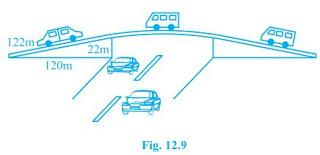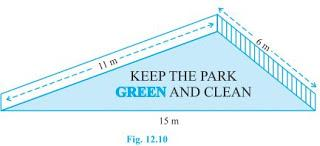# NCERT Solutions For Class 9 Math Chapter – 12 Exercise – 12.1

NCERT Solution For Class 9 Math Chapter – 12 Exercise – 12.1

#### Experts at Sunstarup  have created the NCERT Solutions after extensive search on each topic Students can refer to this study study material to boost their confidence and attempt the second term exam smartly. The concepts are explained with steps, shortcuts to remember formula , tips and tricks to solve the numerical problems wisely and quickly.

Q1.

A traffic signal board indicating ‘SCHOOL AHEAD’ is an equilateral  triangle with side ‘a’ Find the area of the signal board using Heron ‘s Formula If its perimeter is 180 cm , what will be the area of the signal board?

Solution:-

Triangle is an equilateral triangle of side a.

S = a + a + a /2   = 3a/2

Using Heron Formula  we get

Area of triangle = √3a/2 (3a – a)(3a/a – a )(3a/2 – a)

= √3a/2 x a/2 x a/2 x a/2

= √3/4 a2

If perimeter = 180 cm , then side = 180/3

= 60 cm.

Area of triangle = √3/4 x (60)2

= 900√3 cm2.

Q2. The triangular side walls of a flyover have been used for advertisements The sides of the walls are 122 m , 22 m and 120 m The advertisements yield an earning of Rs 5000 per m2 per year A company  hired one of its walls for 3 months How much rent did it pay?

Solution:-S = 122 + 120 + 22 / 2

S = 264/2

S = 132 m

Area = √(132)(132-122)(132-120)(132-22) m2.

= √132 x 10 x 12 x 110 m2.

= √11 x 12 x 10 x 12 x 11 x 10 m2

= 10 x 11 x 12 m2

Rent paid for 3 months = 1320 x 5000 x 3/12

= Rs 16,50,000.

Q3. There is a slide in a park One of its side wall has been painted in some colour with a message “KEEP THE PARK GREEN AND CLEAN “ If the sides of the wall are 15 m 11 m and 6 m find the area painted in colour.Solution:-

S = 15 + 11 + 6/2

S = 32/2

S = 16 m.

Area = √16(16-15)(16-11)(16-6)

= √16 x 1 x 5 x 10

= √800

= 20√2 m2.

Q4. Find the area of a triangle two sides of which are 18 cm and 10 cm and the perimeter is 42 cm.

Solution:-

S = 42/2

S = 21 cm

Area = √21(21-18)(21-10)(21-14)

= √21 x 3 x11 x 7

= 21√11 cm2.Q5. Sides of a triangle are in the ratio of 12:17:25 and its perimeter is 540 cm. Find its area.

Solution:-

12x + 17x + 25x = 540

54x = 540

X = 10

Therefore sides are 120 cm , 170 cm and 250 cm.

Semiperimeter = s = 540/2 = 270 cm

Applying Heron Formula we obtain

Area of the triangle = √270(270-120)(270-170)(270-250)

= √270x150x100x20

= √9x30x30x5x100x2x10

= 3 x 30 x 10 x 10

= 9000 cm2.

Q6. An isosceles triangle has perimeter 30 cm and each of the equal sides is 12 cm Find the area of the triangle .

Solution:-

S = 12 + 12 + 6/2

S = 15

Area = √15(15-12)(15-12)(15-6)

= √15x3x3x9

= 9√15 cm2.